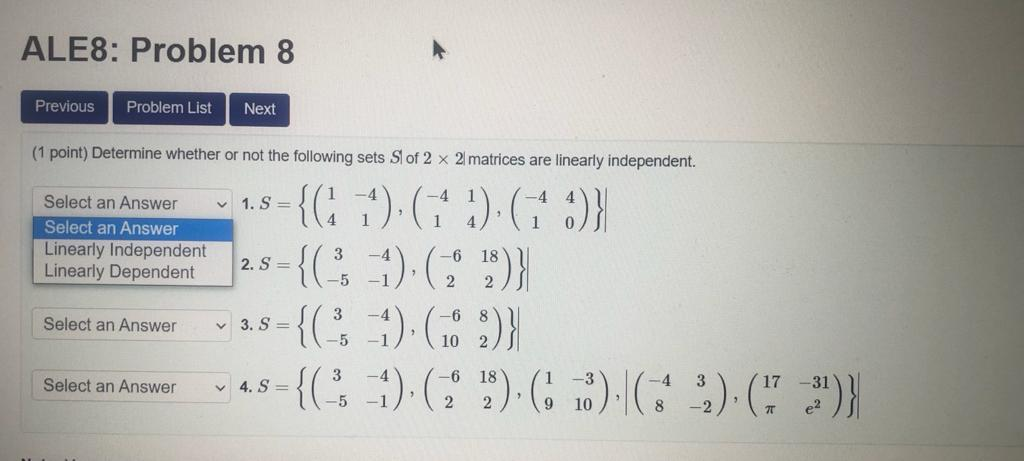### Create an Account

Already have account?

### Forgot Your Password ?

Home / Questions / ALE8: Problem 8 Previous Problem List Next (1 point) Determine whether or not the followin...

# ALE8: Problem 8 Previous Problem List Next (1 point) Determine whether or not the following sets Sl of 2 x 2 matrices are linearly independent. 1. S= Select an Answer Select an Answer Linearly Indepen

ALE8: Problem 8 Previous Problem List Next (1 point) Determine whether or not the following sets Sl of 2 x 2 matrices are linearly independent. 1. S= Select an Answer Select an Answer Linearly Independent Linearly Dependent 2. S = +{C1), (141) (1 :)} ={C-)(3) -{(1) 68 ) -{CS -1) (1) ( ) ( ) (13')} Select an Answer 3. S = Select an Answer 4. S=Apr 15 2021 View more View Less

#### Answer (Solved)Subscribe To Get Solution